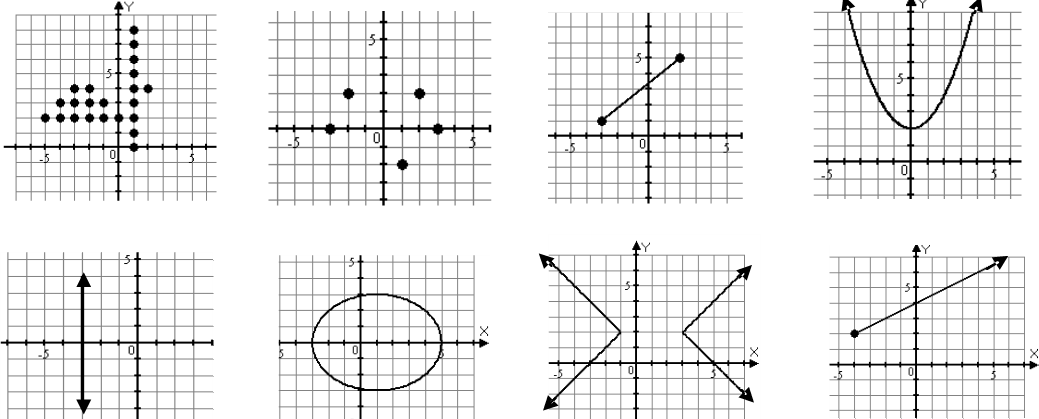# Identifying functions#### Everything You Need in One Place

Homework problems? Exam preparation? Trying to grasp a concept or just brushing up the basics? Our extensive help & practice library have got you covered.#### Learn and Practice With Ease

Our proven video lessons ease you through problems quickly, and you get tonnes of friendly practice on questions that trip students up on tests and finals.#### Instant and Unlimited Help

Our personalized learning platform enables you to instantly find the exact walkthrough to your specific type of question. Activate unlimited help now!

0/2
##### Intros
###### Lessons
1. What is a function?
2. How to do the Vertical Line Test?
1. Equations VS. Functions
0/7
##### Examples
###### Lessons
1. Are the following sets of ordered pairs functions?
1. (4,3), (2,6), (-3,4), (-2,5)
2. (4,5), (4,-3), (2,6), (3,2)
3. (3,6), (2,6), (5,3), (1,2)
2. Which of the following is a function?1. Using the table of values to answer the questions below:
1. X value -5 -3 -1 1 3
Y value 3 1 -1 -2 -3
Express the relations using ordered pairs, mapping notations and determine is it a function
2. X value -5 -3 -1 1 3
Y value 2 2 -1 -2 -3
Express the relations using ordered pairs, mapping notations and determine is it a function
3. X value -5 -5 -1 1 3
Y value 2 3 2 3 -3
Express the relations using ordered pairs, mapping notations and determine is it a function
###### Topic Notes
Bring on more grid papers! In this chapter, we're going to study functions. Functions are notations that tell us what the value of y is for every value of x. By carrying out the vertical line test, we are able to find out the relationships of ordered pairs.
Relations: Sets of ordered pairs ( x , y )

Function: For every value of x, there is a value of y. It will need to pass the vertical line test.

One-To-One Function: For every one value of x, there is only one value of y, and vice versa. It will need to pass both vertical and horizontal line test.

Vertical line Test: A vertical line that intersects the graph of the equation only once when moves from across on the x-axis.